This document provides a brief introduction into the structure of the R package DAIME with an overview of the available functions, some explanations regarding their options, and examples using empirical data.

## Introduction

Changing deposition rates determine how much time it takes to deposit a given thickness of sediment. This determines how diluted or condensed the information contained in this sediment is, and accordingly alters the perception of time and speed when working with the rock record.

The DAIME package allows to

1. Model how changing deposition rates and hiatuses alter the stratigraphic expression of temporal patterns

2. Express data derived from the sedimentary rock record in terms of time

## Available Functions

The version 2.1.3 of the package contains the following functions:

1. `pointtransform` to transform the location of individual points from age/time into stratigraphic height and vice versa. This can also be used to create age models from deposition rates and transform isotope ratios between age/time and height

2. `patterntransform` to transform patterns from age/time into stratigraphic height and vice versa. A pattern can be any grain-based input into the sediment (e.g. fossil occurrences, some geochemical proxies) and rates derived from it (e.g. rate of morphological change or first/last fossil occurrences, both derived from fossil occurrences)

3. `patterntodepositionmodel` to construct a deposition model based on the sedimentary condensation/dilution of known patterns

In addition to that, the following functions are available for backwards compatibility but should nor be used anymore:

• `strattotimepointbin`, `strattotimepointcont`, `timetostratpointbin`, and`timetostratpointcont` (wrappers of `pointtransform`)

• `strattotimeratebin`, `strattotimeratecont`, `timetostratratebin`, and `timetostratratecont` (wrappers of `patterntransform`)

## Direction of Time and Age

The functions `patterntodepositionmodel`, `patterntransform` and `pointtransform` can deal with both time and age. This is determined by the option `timetype`, which is by default set to `timetype="time"`.

## Transformable Data

### Points

Data consisting of individual points can be transformed via the function `pointtransform`. These points can be

• Locations of samples or arbitrary objects found in the sediment (will be transformed into their age/time of deposition)

• Age/time of deposition of any object contained in the sediment (will be transformed into the stratigraphic height where it can be found).

#### Samples associated with measurements

Samples are often associated with measurements performed on this sample (e.g. isotope ratios or Hg content measured on a bivalve). For such samples, only their location needs to be transformed via the DAIME model, the measured values remain unchanged and associated with the sample.

#### Isotope Ratios, Ratios in General and Percentages

In (isotope) ratios, percentages, and ratios in general, values of the numerator and denominator are condensed and diluted equally. This cancels out the effects of sedimentary condensation/dilution on the value (ordinate). However the locations where these values were measured (abscissa) are still affected by condensation/dilution. To account for this, the locations where the ratios were determined need to be transformed via `pointtransform` whilst keeping the values associated with these locations unchanged. For an example, see the help page of `pointtransform`.

### Patterns (Rates)

Patterns can be transformed using the function `patterntransform`. They can be taken as synonymous with rates, and can be subdivided into

• Temporal rates: Any input into the sediment per time unit

• Stratigraphic rates: Any content in the sediment per stratigraphic height

This can for example be

• Fossil rates (e.g. fossil found per meter or shells deposited per year)

• First / last fossil occurrences (but see below)

• Rates of proxies (e.g. Th content per meter/ Th input per time unit)

but also information derived from these rates such as

• Rates of morphological evolution (derived from fossils)

#### First and last fossil occurrences

First and last occurrences are defined post hoc in the section. Due to this they cannot be transformed as a pattern when Hiatuses are present (with no hiatuses around, they can be transformed as any other pattern).

In the presence of hiatuses, simulation approaches or estimates to bracket their position should be used.

### Relation between points and patterns

The relation between patterns and points is similar to the relation between a distribution and a sample. One is the abstract description (pattern) while one is the realization (point)

## Deposition models

Deposition models are central to the DAIME package and describe how sediment input into the system changes through time. There are three ways to hand a deposition model over to the functions `pointtransform` and `patterntransform`:

• as age model

• as piecewise linear deposition rate

• as binned deposition rate.

By default, the deposition model is assumed to be a piecewise linear deposition rate. This can be adjusted using the `depositionmodel` option.

### Age models

Age models assign a stratigraphic height its time/age of deposition and (equivalently) an age/time the stratigraphic height that was deposited at said age.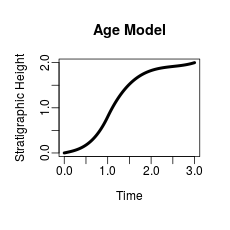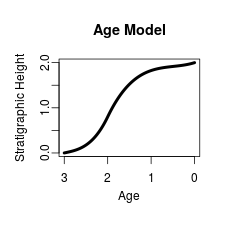### Deposition Rates

Deposition rates describe the sediment input into a system through time. They can be handed over either as piecewise linear deposition rate by setting `depositionmodel` to `piecewise linear deposition rate` or as binned deposition rate by setting `depositionmodel` to `binned deposition rate`.

#### Piecewise linear deposition rates

Piecewise linear deposition rates are described by two vectors of the same length, `xdep` and `ydep`. `ydep[i]` gives the deposition rate at the time/age/height `xdep[i]`, In between the values, the deposition rates are interpolated linearly: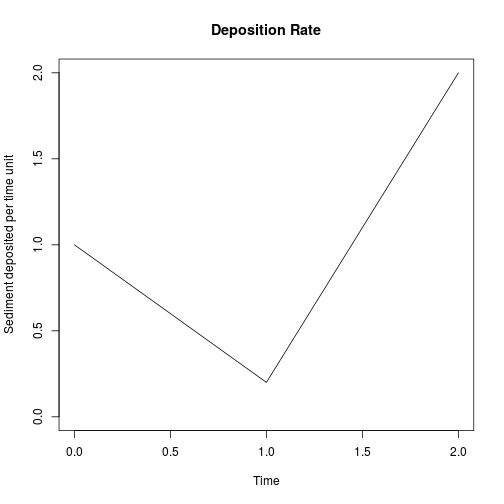So as a function, the deposition rate is given by `depositionrate=approxfun(xdep,ydep)`

#### Binned depositon rates

Binned deposition rates are described by two vectors `xdep` and `ydep`. The deposition rate in the interval in time/age/height) between `xdep[i]` and `xdep[i+1]` is given by `ydep[i]`. Accordingly `ydep` is one entry shorter than `xdep`.

#### Inverse deposition rates and units

For the transformation from height to time, the additional option `unit` is available. This is since deposition rates in the section can be described in two ways:

1. Either they give a description of the deposition rates with which the heights in the section were deposited

2. Or they describe how much time is “contained” in sediment (“inverse” deposition rate).

Setting `unit` to `sediment per time` and the functions will interpret the deposition rate described by `xdep` and `ydep` in the first way. Setting is to `time per sediment` will lead to the functions interpreting the deposition rates in the second way.

The default setting is `unit='sediment per time'`. As an example of the effects of this option, the following stratigraphic pattern is transformed into time: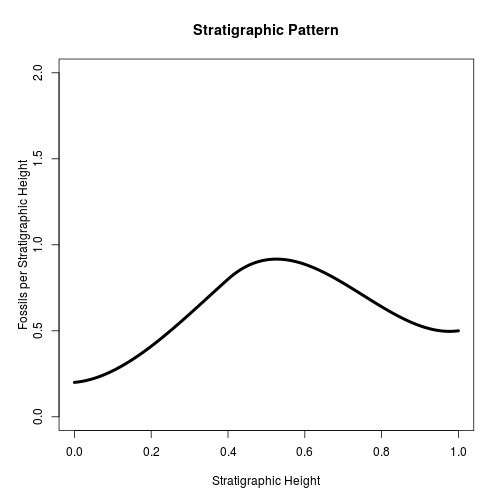First, using the default option of “unit='sediment per time'”, the deposition rate is given at the left (note the y axis), and the result of the transformation is given at the right.

``````#> Loading required package: DAIME
``````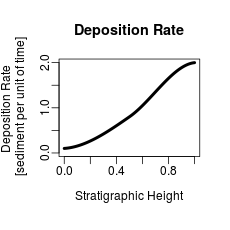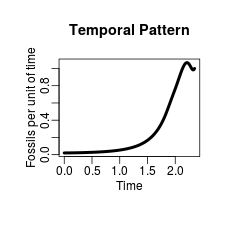Setting “unit='time per sediment'”, the inverse deposition rate is given at the left (note the y axis), and the result of the transformation is given at the right.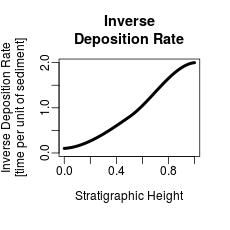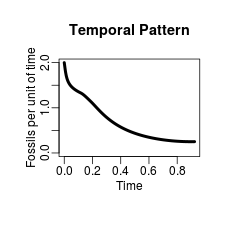Note how different the results are! This is since a high deposition rate corresponds to a high dilution, whereas a high inverse deposition rate corresponds to high condensation.

### Creating age models from the condensation/dilution of patterns

The function `patterntodepositionmodel` creates an age model that condenses/dilutes a given temporal pattern into a given stratigraphic pattern and vice versa. For examples see example 2 and 3 below or check `?patterntodepositionmodel`

### Hiatuses, Erosion, and Nondeposition

The handling of hiatuses, erosion, and nondeposition depends on the direction of transformation.

For the transformation from section to time, i.e. using the option `direction="height to time"`, hiatuses can be inserted at any stratigraphic height using the `hiatuslist` option. For details see the corresponding help pages.

For the transformation from time to the section, i.e. using the option `direction="time to height"`, hiatuses, erosion, and nondeposition can be modeled via deposition rates that are zero (nondeposition) or negative (erosion) or as time intervals where the age model is decreasing.

Hiatuses are destructive in this implementation of the DAIME model. Anything being deposited right at a hiatus or during an interval of nondeposition or erosion will be destroyed by the transformation.

## Examples

### Example 1: The K/Pg boundary on Seymour Island, Antarctica

The DAIME Package automatically loads some age models and extinction hypotheses for the K/Pg boundary

``````require(DAIME)
#Check help page for data sources and information on the age models:
?SeymourIslandAgeModels

my.agemodel=SeymourIslandAgeModels\$C #Select one of the age models
#plot age model
plot(my.agemodel\$age,my.agemodel\$height,
xlab='Age [Ma]',ylab = 'Height [m]',
xlim=rev(range(my.agemodel\$age)),type='l')
``````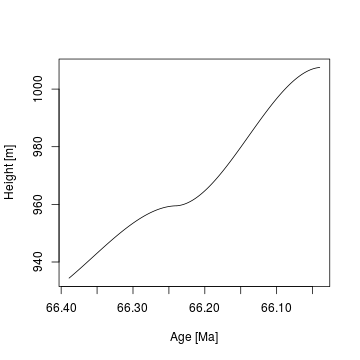``````## Get extinction hypotheses
#Check help page for data sources and information on the last occurrences:
?KPgLastOccurrences

#Select extinction hypotheses with intermediate influence of the Deccan trapps
temp.pat=KPgLastOccurrences\$Intermediate.Deccan
temp.pat\$parameters #check description of extinction hypotheses
#>  "Extinction pulse at K/Pg boundary combined with extinction interval from fig. 5 from Tobin (2017), which was transformed into time assuming constant deposition. Rate of fossil occurrences is 40 fossils per ma, relative contribution of the early extinction interval to the total number of extinctions is 20 %. Pattern normalized to be a probability density function."
#Plot extinction hypotheses (=last occurrences)
plot(temp.pat\$age,temp.pat\$val,
xlab='Age [Ma]',ylab='Intensity [Last occurrences per Myr]',
xlim=rev(range(temp.pat\$age)),type='l')
``````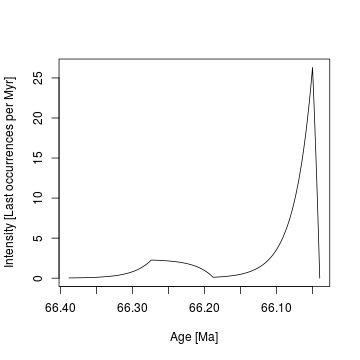#### Transforming (piecewise linear) temporal patterns

Using the function `patterntransform`, the extinction hypotheses in time can be transformed into stratigraphic height. Here, the previously selected age model is used as the deposition model.

``````strat.pat=patterntransform(xdep=my.agemodel\$age,ydep=my.agemodel\$height,
xpat=temp.pat\$age,ypat=temp.pat\$val,
direction = 'time to height',
depositionmodel = 'age model',
patternmode = 'piecewise linear',
timetype = 'age')
strat.pat\$report #What was done?
#>  "Transformed piecewise linear pattern from  time to height using a age model as deposition model. 0 hiatus(es) in the section. Interpreted input as age"
#Plot last occurrences in stratigraphic height
plot(strat.pat\$height,strat.pat\$val,
xlab='Stratigraphic height [m]',ylab='Intensity [Last occurrences per m]',
type='l')
``````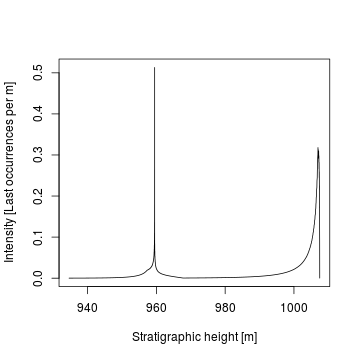#### Transforming points

The function `pointtransform` allows to transform individual points. To demonstrate this, the sampling bins from Macellari (1984) are transformed into time to study their temporal resolution.

``````##Sampling bins are automatically loaded with the DAIME package
#Check help page for data sources and information on the sampling bins:
?SeymourIslandBins

samplingbins=SeymourIslandBins\$Macellari.1984.Section.D.
trans.bins=pointtransform(points=samplingbins,
xdep=my.agemodel\$height,ydep=my.agemodel\$age,
direction='height to time',
depositionmodel = 'age model',
timetype = 'age')
trans.bins\$age #ages corresponding to the limits of the sampling bins
#>        NA       NA       NA       NA       NA 66.23900 66.18522 66.04000
#Note that points that are outside the deposition model are returned as NA !
abs(diff(trans.bins\$age)) #Duration of bins in Myr.
#>        NA       NA       NA       NA       NA 0.053779 0.145221
#The bin before the K/Pg contains almost 0.15 Myr
``````

#### Transforming (binned) stratigraphic patterns

The function `patterntransform` can also be used to transform rates such as fossil abundances from the section into time

``````#Sampling relevant sampling bins from Witts et al. (2016)
WittsBins=SeymourIslandBins\$Witts.et.al.2016.Section.A
WittsBins=WittsBins[WittsBins>=min(my.agemodel\$height) &WittsBins<=max(my.agemodel\$height)]
#No. of fossil finds of Maorites densicostatus in section A from WItts et al. (2016)
Maorites.densicostatus=c(7,7,29,1,0,1,6)
#Fossils per meter
Foss.Rate=Maorites.densicostatus/diff(WittsBins)
#Plot fossils per meter
plot(approx(x=WittsBins,y=c(Foss.Rate,0),method='constant',xout=my.agemodel\$height),
xlab='Stratigraphic height [m]',
ylab = 'Fossil abundance [Fossils per m]',main='Maorites densicostatus',
type='l')
``````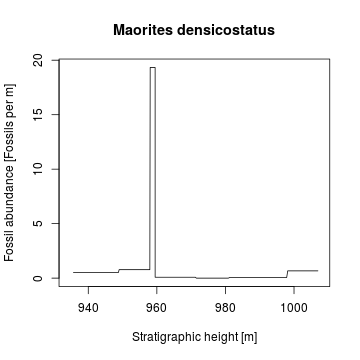This binned stratigraphic pattern can be transformed into a temporal pattern:

``````temp.pat=patterntransform(xdep=my.agemodel\$height,ydep=my.agemodel\$age,
xpat=WittsBins,ypat=Foss.Rate,
depositionmodel = 'age model',direction='height to time',
patternmode = 'binned',
timetype = 'age')
plot(temp.pat\$age,temp.pat\$val,
xlab='Age [ma]',ylab='Fossil abundance [Fossils per Myr]',
main='Maorites densicostatus', xlim = rev(range(my.agemodel\$age)),
type='l')
``````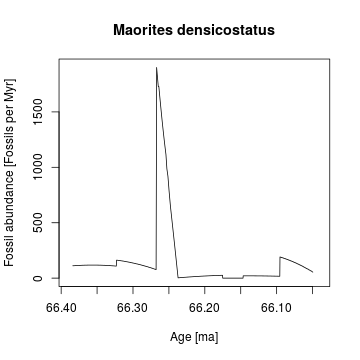### Example 2: The Oceanic Anoxic Event 1d at the Col de Palluel, France

Data for this Example is from Bornemann et al (2005) and is automatically loaded with the DAIME package.

``````#Check help page for data sources and information on the data:
?ColDePalluel
#Use the following abbreviations
palyn=ColDePalluel\$palynomorphs
geochem=ColDePalluel\$geochemistry
``````

#### Construction age models from dilution/condensation of sediment contents

First, an age model is build on the assumption of constant input of saccate pollen:

``````my.agemodel=patterntodepositionmodel(xheight=palyn\$Depth..m.,
yheight = palyn\$Pollen.sac....,
timetype = 'time')
plot(my.agemodel\$time,my.agemodel\$height,
xlab='Relative Time',ylab='Stratigraphic Height [m]',
type='l')
lines(range(my.agemodel\$time),range(my.agemodel\$height),lty=3)
legend('topleft',legend='Age Model',lty=1,lwd=1)
``````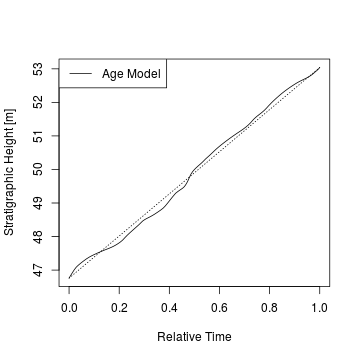#### Transforming (piecewise linear) stratigraphic patterns

Using this age model, the number of dinoflagellate cysts is transformed into time:

``````#Transform dinoflagellate cysts into relative time
temp.pat=patterntransform(xdep=my.agemodel\$height,ydep=my.agemodel\$time,
xpat=palyn\$Depth..m.,ypat=palyn\$Dinofl.cyst....,
direction='height to time',timetype = 'time',
depositionmodel = 'age model')
plot(palyn\$Depth..m.,palyn\$Dinofl.cyst....,
xlab='Stratigraphic Height [m]',ylab='Dinoflagellate cysts [counts]',
type='l')
``````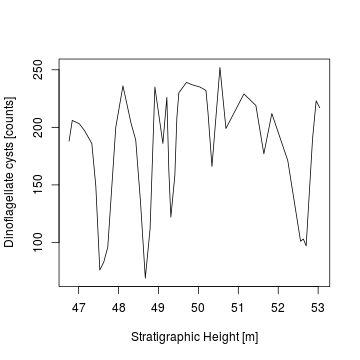``````plot(temp.pat\$time,temp.pat\$val,
xlab='Relative Time', ylab='Dinoflagellate cysts [counts]',
type='l')
``````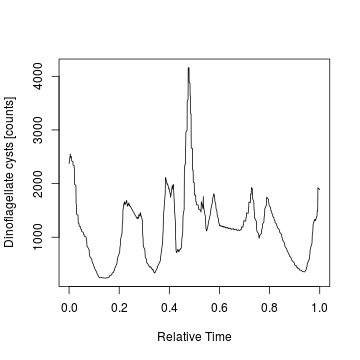#### Identifying points in time and stratigraphic height

The spike in Dinoflagellate cysts in time is curious, so let's find out to what stratigraphic height it corresponds:

``````#Time of spike
spike.time=temp.pat\$time[which(temp.pat\$val==max(temp.pat\$val))]
#Transform time of spike into height
poi=pointtransform(points=spike.time,
xdep=my.agemodel\$time,ydep=my.agemodel\$height,
direction='time to height',
depositionmodel = 'age model')
#stratigraphic height of spike
poi\$height
#>  49.70198
#get lithology closest to the spike
geochem\$Lithology[which(abs(geochem\$Depth..m.-poi\$height)==min(abs(geochem\$Depth..m.-poi\$height)))]
#>  marlstone/concr.
#> 6 Levels: black shale clayey marlstone limestone? limy marlstone ... marlstone/concr.
``````

#### Transforming Isotope values

Last, the carbon isotope values are transformed into time. For this, only the heights of the locations are transformed into time while the isotope values remain unchanged

``````#get times of deposition for geochemnistry data
sampletimes.geochem=pointtransform(points=geochem\$Depth..m.,xdep=my.agemodel\$height,
ydep=my.agemodel\$time,
direction='height to time',
depositionmodel = 'age model',
timetype = 'time')

#transform delta13 C into time
plot(sampletimes.geochem\$height, geochem\$delta13C.carb....PDB.,
xlab='Stratigraphic Height [m]', ylab = "delta13C [per mil]",
type='l')
``````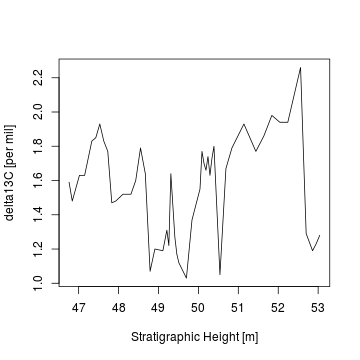``````plot(sampletimes.geochem\$time, geochem\$delta13C.carb....PDB.,
xlab = 'Relative Time',ylab='delta13C [per mil]',
type='l')
``````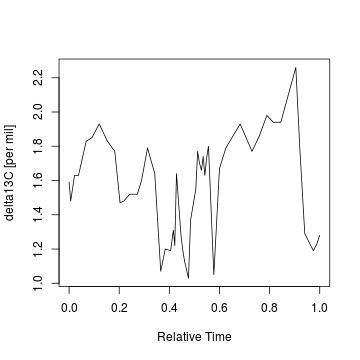Note that there is only a weak squeezebox effect on the isotope curves since there are no intervals where changes in isotope values coincide with sedimentary condensation/dilution

### Example 3: Age models and sediment accumulation rates in recent environments at Lake Superior

To demonstrate the reconstruction of age models from 210Pb values using the DAIME model, I use data from core BH03-3 in O'Beirne et al. (2017). This data is is automatically loaded with the DAIME package. Sediment accumulation rates correspond to the slope of the age models constructed in these examples.

``````#Check help page for data sources and information on the data:
?LakeSuperior
#The stratigraphic pattern is given by the measured excess lead
plot(LakeSuperior\$BH03_3\$Depth..m.,LakeSuperior\$BH03_3\$X210Pb.xs..Bq.kg.,
main='Stratigraphic Pattern')
``````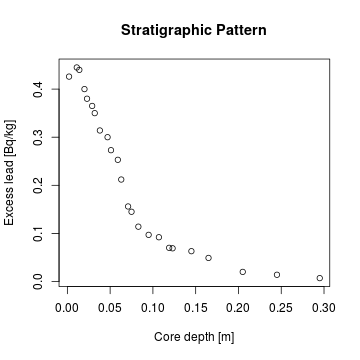``````#The temporal pattern is excess lead content in the sediment
#this will follow an exponential decay
dec.const=log(2)/22.3 #decay constant of 210Pb
temp.pat.x=seq(0,300,by=0.1)
temp.pat.y=exp(-dec.const*temp.pat.x)
plot(temp.pat.x,temp.pat.y,
xlab = 'Sediment Age [years]',
ylab='Proportion of initial Excess lead remaining in sediment',
main='Temporal pattern',
type='l')
``````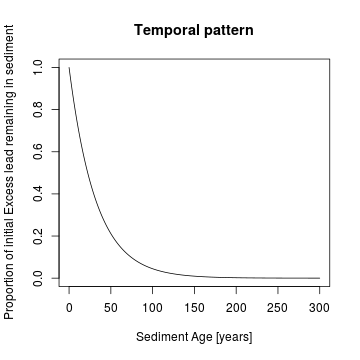Since both stratigraphic pattern and temporal pattern are known, the function 'patterntodepositionmodel' can be used to create an age model. Since the stratigraphic pattern determines the amount of excess 210Pb in the system, the option 'rescalefor' is set to 'stratigraphic pattern'

``````agemodel=patterntodepositionmodel(xheight=LakeSuperior\$BH03_3\$Depth..m.,
yheight = LakeSuperior\$BH03_3\$X210Pb.xs..Bq.kg.,
xage = temp.pat.x,yage=temp.pat.y,
timetype = 'time',
rescalefor = 'stratigraphic pattern')
#note that "timetype" is set to 'time', and not 'age'.
#This is since although we are interested in age, it is examined from youngest to oldest (in reverse order)
plot(agemodel\$time,agemodel\$height,
xlab='Years before present',
ylab='Core depth [m]',
main='Age Model',
lwd=3,type='l')
lines(x=1000*LakeSuperior\$BH03_3\$Age.model..ka., #Age model from O\'Beirne et al. (2017) is given in kyr
y=LakeSuperior\$BH03_3\$Depth..m.,
lty=3)
legend('bottomright',
lwd=c(3,1),lty=c(1,3),
legend=c('DAIME','O\'Beirne et al. (2017)'))
``````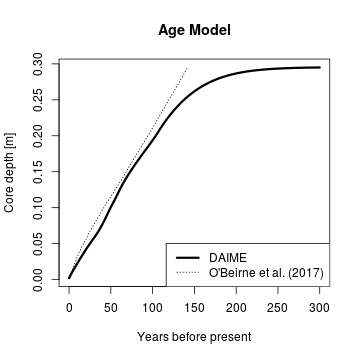It is clearly visible how the agemodel becomes more unrealistic down core. This is since in these depths, small changes 210Pb correspond to huge differences in age.

The DAIME model also allows to build age models in situations where 210Pb input changes through time:

``````#Assume a changing input of 210Pb:
Pb.input=splinefunH(x=c(0,89,300),y=c(1,5,1),m=c(0.1,-0.1,-0.01))(temp.pat.x)
plot(temp.pat.x,Pb.input,
xlab='Years before present', ylab='210Pb input',
ylim=c(0,6),type='l')
``````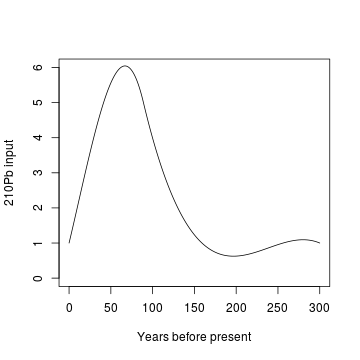``````#multiplying the input with the curve from the radioactive decay yields the temporal pattern:
temp.pat.y2=Pb.input*temp.pat.y
plot(temp.pat.x,temp.pat.y2,
xlab='Sediment Age',ylab='210 Pb content of Sediment',
main='Temporal pattern',
type='l')
``````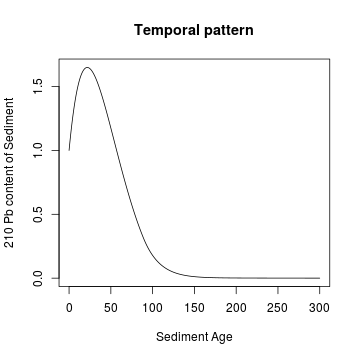``````#This pattern can now again be used to create an age model
agemodel=patterntodepositionmodel(xheight=LakeSuperior\$BH03_3\$Depth..m.,
yheight = LakeSuperior\$BH03_3\$X210Pb.xs..Bq.kg.,
xage = temp.pat.x,
yage=temp.pat.y2,
timetype = 'time',
rescalefor = 'stratigraphic pattern')
#
plot(agemodel\$time,agemodel\$height,
xlab='Years before present', ylab='Core depth [m]',
main='Age Model',
lwd=3, xlim=c(0,150),type='l')
``````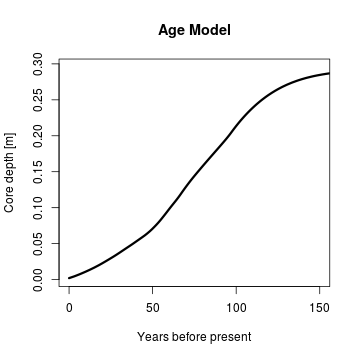## References

Bornemann, A et al. (2005): Reconstruction of short-term palaeoceanographic changes during the formation of the Late Albian Niveau Breistroffer black shales (Oceanic Anoxic Event 1d, SE France). Journal of the Geological Society of London, 162(4), 623-639, https://doi.org/10.1144/0016-764903-171

Witts, James D., et al. “Macrofossil evidence for a rapid and severe Cretaceous/Paleogene mass extinction in Antarctica.” Nature communications 7 (2016): 11738. https://doi.org/10.1038/ncomms11738

O'Beirne, MD et al. (2017): Anthropogenic climate change has altered primary productivity in Lake Superior. Nature Communications, 8, 15713, https://doi.org/10.1038/ncomms15713

Hohmann, Niklas. 2018. Quantifying the Effects of Changing Deposition Rates and Hiatii on the Stratigraphic Distribution of Fossils. https://doi.org/10.13140/RG.2.2.23372.51841